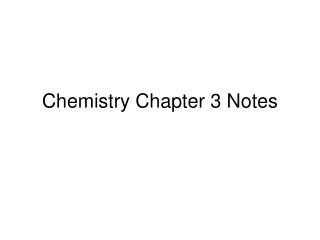DownloadDownload PresentationChemistry Chapter 3 Notes

# Chemistry Chapter 3 Notes

Download Presentation## Chemistry Chapter 3 Notes

- - - - - - - - - - - - - - - - - - - - - - - - - - - E N D - - - - - - - - - - - - - - - - - - - - - - - - - - -
##### Presentation Transcript

1. Chemistry Chapter 3 Notes

2. Using and Expressing Measurements • measurement • a quantitative description that includes both a number and a unit (3.1)

3. Measurements are fundamental to the experimental sciences. For that reason, it is important to be able to make measurements and to decide whether a measurement is correct.

4. scientific notation • an expression of numbers in the form m× 10n where mis equal to or greater than 1 and less than 10, and nis an integer(3.1)

5. For example, the number 602,000,000,000,000,000,000,000written in scientific notation is 6.02 × 1023. The coefficient in this number is 6.02. In scientific notation, the coefficient is always a number equal to or greater than one and less than ten. The power of 10, or exponent, in this example is 23.

6. Accuracy, Precision, and Error • Accuracy • the closeness of a measurement to the true value of what is being measured(3.1)

7. Precision • describes the closeness, or reproducibility, of a set of measurements taken under the same conditions(3.1)

8. To evaluate the accuracy of a measurement, the measured value must be compared to the correct value. To evaluate the precision of a measurement, you must compare the values of two or more repeated measurements.

9. Look at Figure 3.2 as you consider the following outcomes. • All of the darts land close to the bull’s-eye and to one another. Closeness to the bull’s-eye means that the degree of accuracy is great. Each dart in the bull’s-eye corresponds to an accurate measurement of a value. Closeness of the darts to one another indicates high precision.

10. All of the darts land close to one another but far from the bull’s-eye. The precision is high because of the closeness of grouping and thus the high level of reproducibility. The results are inaccurate, however, because of the distance of the darts from the bull’s-eye.

11. The darts land far from one another and from the bull’s-eye.The results are both inaccurate and imprecise.

12. Determining Error • Note that an individual measurement may be accurate or inaccurate

13. accepted value • a quantity used by general agreement of the scientific community(3.1) • experimental value • a quantitative value measured during an experiment (3.1)

14. error • the difference between the accepted value and the experimental value (3.1) • Error = experimental value − accepted value

15. percent error • the percent that a measured value differs from the accepted value(3.1)

16. Significant Figures in Measurements • significant figures • all the digits that can be known precisely in a measurement, plus a last estimated digit(3.1)

17. Measurements must always be reported to the correct number of significant figures because calculated answers often depend on the number of significant figures in the values used in the calculation.

18. Significant Figures in Calculations • In general, a calculated answer cannot be more precise than the least precise measurement from which it was calculated

19. Once you know the number of significant figures your answer should have, round to that many digits, counting from the left. If the digit immediately to the right of the last significant digit is less than 5, it is simply dropped and the value of the last significant digit stays the same. If the digit in question is 5 or greater, the value of the digit in the last significant place is increased by 1.

20. Addition and Subtraction • The answer to an addition or subtraction calculation should be rounded to the same number of decimal places (not digits) as the measurement with the least number of decimal places.

21. Multiplication and Division • In calculations involving multiplication and division, you need to round the answer to the same number of significant figures as the measurement with the least number of significant figures.

22. Key Concept How do measurements relate to experimental science?Hint • (10) Key Concept How are accuracy and precision evaluated?Hint • (11) Key Concept Why must a given measurement always be reported to the correct number of significant figures?Hint

23. 12) Key Concept How does the precision of a calculated answer compare to the precision of the measurements used to obtain it?Hint • (13)A technician experimentally determined the boiling point of octane to be 124.1°C. The actual boiling point of octane is 125.7°C. Calculate the error and the percent error.

24. 14)Determine the number of significant figures in each of the following. • 11 soccer players • 0.070 020 meter • 10,800 meters • 5.00 cubicmeters

25. (15)Solve the following and express each answer in scientific notation and to the correct number of significant figures. • (5.3 × 104) + (1.3 × 104) • (7.2 × 10−4) ÷ (1.8 × 103) • 104× 10−3× 106 • (9.12 × 10−1) − (4.7 × 10−2) • (5.4 × 104) × (3.5 × 109)